# Noetherian ring

(diff) ← Older revision | Latest revision (diff) | Newer revision → (diff)

left (right)

A ringsatisfying one of the following equivalent conditions:

1)is a left (or right) Noetherian module over itself;

2) every left (or right) ideal inhas a finite generating set;

3) every strictly ascending chain of left (or right) ideals inbreaks off after finitely many terms.

An example of a Noetherian ring is any principal ideal ring, i.e. a ring in which every ideal has one generator.

Noetherian rings are named after E. Noether, who made a systematic study of such rings and carried over to them a number of results known earlier only under more stringent restrictions (for example, Lasker's theory of primary decompositions).

A right Noetherian ring need not be left Noetherian and vice versa. For example, letbe the ring of matrices of the formwhereis a rational integer andandare rational numbers, with the usual addition and multiplication. Thenis right, but not left, Noetherian, since the left ideal of elements of the formdoes not have a finite generating set.

Quotient rings and finite direct sums of Noetherian rings are again Noetherian, but a subring of a Noetherian ring need not be Noetherian. For example, a polynomial ring in infinitely many variables over a field is not Noetherian, although it is contained in its field of fractions, which is Noetherian.

Ifis a left Noetherian ring, then so is the polynomial ring. The corresponding property holds for the ring of formal power series over a Noetherian ring. In particular, polynomial rings of the form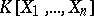or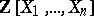, whereis a field andthe ring of integers, and also quotient rings of them, are Noetherian. Every Artinian ring is Noetherian. The localization of a commutative Noetherian ringrelative to some multiplicative systemis again Noetherian. If in a commutative Noetherian ring,is an ideal such that no element of the form, where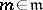, is a divisor of zero, then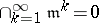. This means that any such ideal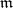defines ona separable-adic topology. In a commutative Noetherian ring every ideal has a representation as an incontractible intersection of finitely many primary ideals. Although such a representation is not unique, the number of ideals and the set of prime ideals associated with the given primary ideals are uniquely determined.

How to Cite This Entry:
Noetherian ring. Encyclopedia of Mathematics. URL: http://encyclopediaofmath.org/index.php?title=Noetherian_ring&oldid=18864
This article was adapted from an original article by L.V. Kuz'min (originator), which appeared in Encyclopedia of Mathematics - ISBN 1402006098. See original article